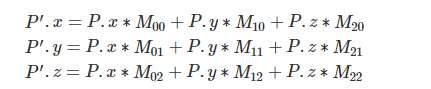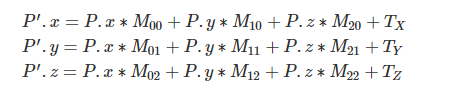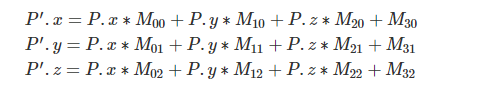•图像处理
• 问题： 请将一个具有n个元素的一维向量向左旋转i个位置。例如i = 3，那么向量abcdefgh旋转之后得到向量...旋转向量X实际上就是将向量ab的两个部分交换为向量ba，我们先从ab开始，转置a得到aTb，再转置b得到aTbT，然
问题：

请将一个具有n个元素的一维向量向左旋转i个位置。例如i = 3，那么向量abcdefgh旋转之后得到向量defghabc。

方案一：

旋转向量X实际上就是将向量ab的两个部分交换为向量ba，我们先从ab开始，转置a得到aTb，再转置b得到aTbT，然后转置aTbT，得到(aTbT)T，即ba，则有，  reverse(0, i - 1);     // cbadefgh reverse(i, n - 1);     // cbahgfed reverse(0, n - 1);   // defghabc

实现例程：

template<typename T>
void reverse(vector<T> &m, int x, int y)
{
T tmp;
while (x < y)
{
tmp = m[x];
m[x] = m[y];
m[y] = tmp;
x++;
y--;
}
}

template<typename T>
// 将abcdefgh变为defghabc， i = 3
void move(vector<T> &m, int i)
{
int n = m.size();
reverse(m, 0, i - 1);
reverse(m, i, n - 1);
reverse(m, 0, n - 1);
}

int _tmain(int argc, _TCHAR* argv[])
{
int a[] = {123, 24, 23, 4, 523, 5, 4, 6, 43, 5, 23, 65, 234};
vector<int> m(a, a + 13);
move(m, 3);

for (vector<int>::iterator iter = m.begin(); iter != m.end(); iter++)
cout << *iter << " ";

getchar();
return 0;
}


输出：

4， 523， 5， 4， 6， 43， 5， 23， 65， 234， 123， 24， 23

展开全文iterator
• 平面向量的数量积平移的综合练习课[精选].doc
• 用4*4的矩阵来描述向量与点...　那么点与向量就没法区别对待，但是点平移与向量平移是不同的，点平移要改变，向量 　平移不变，所以，需要扩展为4*4的。 　2.如果使用4*4的矩阵来描述一个点 or向量： 　点A = [x,...

用4*4的矩阵来描述向量与点：
1.为什么要用4*4的矩阵，而不是3*3的矩阵呢？
因为在3D世界中，描述一个点至少需要3个维度，如果使用3个维度来描述向量或者点，
那么点与向量就没法区别对待，但是点平移与向量平移是不同的，点平移要改变，向量
平移不变，所以，需要扩展为4*4的。
2.如果使用4*4的矩阵来描述一个点 or向量：
点A = [x,y,z,w]，向量B = [a,b,c,w]
由以前的篇幅可知，一个矩阵乘以单位矩阵结果不变，那么我们用单位矩阵C来乘以 A /B.
[x,y,z,w] *[1,0,0,0 ]   x*1+y*0+z*0+w*0 =x
0,1,0,0    x*0+y*1+z*0+w*0 =y
0,0,1,0    x*0+y*0+z*1+w*0 =z
0,0,0,1    x*0+y*0+z*0+w*1 =w
同时，将点A移动一段距离，假设移动向量N = [α,β,γ,δ]，根据以前知识可知，结果为两个向量
相加，A+N =[x+α,y+β,z+γ,w+1]
那么乘以这样的向量N'
[x,y,z,w] *[1,0,0,0 ]   x*1+y*0+z*0+w*α =x+w*α
0,1,0,0    x*0+y*1+z*0+w*β =y+w*β
0,0,1,0    x*0+y*0+z*1+w*γ =z+w*γ
α,β,γ,1    x*0+y*0+z*0+w*1 =w
从此次可知，只要w =0，那么位移一段距离也不会改变A，如果w =1，那么就会改变位置，所以可以描述
向量。

转载于:https://www.cnblogs.com/leiGameDesigner/p/8371642.html
展开全文• 在优化过程中,求解出来的se3小量前三列并不是平移向量,因此需要转换一下: se3.cpp源码: SE3 SE3::exp(const Vector6d & update) { Vector3d upsilon = update.head<3>(); Vector3d omega = update.tail&...
在优化过程中,求解出来的se3小量前三列并不是平移向量,因此需要转换一下: se3.cpp源码:
SE3 SE3::exp(const Vector6d & update)
{
Vector3d omega = update.tail<3>();

double theta;
SO3 so3 = SO3::expAndTheta(omega, &theta);

Matrix3d Omega = SO3::hat(omega);
Matrix3d Omega_sq = Omega*Omega;
Matrix3d V;

if(theta<SMALL_EPS)
{
V = so3.matrix();
//Note: That is an accurate expansion!
}
else
{
double theta_sq = theta*theta;
V = (Matrix3d::Identity()
+ (1-cos(theta))/(theta_sq)*Omega
+ (theta-sin(theta))/(theta_sq*theta)*Omega_sq);
}
return SE3(so3,V*upsilon);
}

自己改写后的代码,只求平移向量:
 Eigen::Matrix3d expAndTheta(const Eigen::Vector3d & omega, double * theta)
{
double SMALL_EPS = 1e-10;
*theta = omega.norm();
double half_theta = 0.5*(*theta);

double imag_factor;
double real_factor = cos(half_theta);
if((*theta)<SMALL_EPS)
{
double theta_sq = (*theta)*(*theta);
double theta_po4 = theta_sq*theta_sq;
imag_factor = 0.5-0.0208333*theta_sq+0.000260417*theta_po4;
}
else
{
double sin_half_theta = sin(half_theta);
imag_factor = sin_half_theta/(*theta);
}

Eigen::Matrix3d rotation_matrix;
rotation_matrix= Eigen::Quaterniond(real_factor,
imag_factor*omega.x(),
imag_factor*omega.y(),
imag_factor*omega.z()).matrix();
return rotation_matrix;
}

Eigen:: Matrix3d hatmat(const Eigen::Vector3d & v)
{
Eigen::Matrix3d Omega;
Omega <<  0, -v(2), v(1)
,v(2), 0, -v(0)
,-v(1),v(0), 0;
return Omega;
}

Eigen::Vector3d calTl(const Eigen::Matrix<double,6,1>& update)
{
double SMALL_EPS = 1e-10;
Eigen::Vector3d omega = update.tail<3>();

// Eigen::Vector3d upsilon {update,update,update};
// Eigen::Vector3d omega {update,update,update};

//double theta = omega.norm();
double theta ;
Eigen:: Matrix3d so3 = expAndTheta(omega, &theta);

Eigen::Matrix3d Omega = hatmat(omega);
Eigen::Matrix3d Omega_sq = Omega*Omega;
Eigen::Matrix3d V;

if(theta<SMALL_EPS)
{
V = so3;
//Note: That is an accurate expansion!
}

else
{
double theta_sq = theta*theta;
V = (Eigen::Matrix3d::Identity()
+ (1-cos(theta))/(theta_sq)*Omega
+ (theta-sin(theta))/(theta_sq*theta)*Omega_sq);
}
return V*upsilon;
}

展开全文sophus exp
• 对一个点或者向量进行旋转的时候，做法是让这个点（或向量，为了叙述方便，以下只谈论点的情况，向量的情况也是一样的），乘以一个矩阵M，根据之前的知识（参考上一篇blog:...要让P点能够平移，先要思考什么...

对一个点或者向量进行旋转的时候，做法是让这个点（或向量，为了叙述方便，以下只谈论点的情况，向量的情况也是一样的），乘以一个矩阵M，根据之前的知识（参考上一篇blog: https://www.cnblogs.com/heben/p/9182090.html），矩阵M是一个3X3的方阵。

于是点P经过旋转之后，新点P'的坐标计算公式如下：然而这只是旋转。要让P点能够平移，先要思考什么是平移，其实平移就是对P点的每一个坐标加上一个数字，所以带上平移的功能之后，新点P'的坐标应该是下面这样：为了将Tx的信息能够存入M矩阵，scratchapixel介绍的方式是，为M矩阵新增一行，将Tx存储为M30，将Ty存储为M31，将Tz存储为M32
于是P'坐标的计算方式就变成：这时M已经成为了一个4X3矩阵，为了满足矩阵的乘法，显然P点也需要改写，写成P(x, y ,z, 1)这样的形式。不过在实际编码中，并不会真的给坐标加上一个第四维度的1，而只是假装那里有个1。点P形式为(x, y, z ,1)的时候，我们称这样的点为同质点(homogeneous point)
最后，CG中常用的M矩阵式4X4的。但这需要了解更多的知识，现在只需要知道，矩阵M由4x3变成4x4，就是新增一列，大部分情况下这一列的数值是(0, 0, 0, 1)

转载于:https://www.cnblogs.com/heben/p/9193624.html
展开全文• 根据 三点在两个坐标系下的坐标，建立两个坐标系之间的旋转矩阵R和平移向量T，适用场景：...以其中一点建立 世界坐标系，该点在相机坐标系中的坐标是 世界坐标系到坐标系的平移向量（这些描述都是以相机坐标系为基准）计算机视觉
• 把旋转向量转换成旋转矩阵SO(3)，使用罗德里格斯公式进行转换 需要注意到的是solvepnp函数解出来的是旋转向量r,将r转换成这种型式r=[\theta]
• 然后对应的有平移向量tvec如下 tvec=[0.005806541820330855;  0.001584643814482065;  0.003887003832579859] 通过下一段代码就能的到对应的旋转矩阵SE(3)了 Eigen::Isometry3d T = Eigen::Isometry3d::...
• 图像先绕坐标原点旋转，旋转之后图像的中心点到了另一个位置。 旋转前后图像的中心点就不在一个位置了，为了让它们在一个位置上，需要再平移...图像中心点旋转后的坐标减去旋转前的坐标就是转换矩阵中的平移向量了。
• 复习五——平面向量的数量积的坐标表示、平移[精选].doc
• ## 平面向量

千次阅读 2015-07-31 23:20:59
平面向量是在二维平面内既有方向(direction)又有大小(magnitude)的量, 物理学中也称作矢量, 与之相对的是只有大小, 没有方向的数量(标量). 平面向量用小写加粗的字母a, b, c表示; 也可以用表示向量的有向线段的...
• 有的时候变换可能比较复杂，比如平移之后又旋转，旋转之后又平移，又缩放。 直接用公式计算，不但复杂，而且效率低下。这时可以借助变换矩阵和矩阵乘法，将多个变换合成一个。 最后只要用一个矩阵对每个点做一次...缩放
• 8.4.2.2_利用最小平移向量使两个物体粘在一起<!DOCTYPE html> 利用最小平移向量使两个物体粘在一起 body{ background: #fff;
• 平移向量 将一个具有n个元素的一维向量向左旋转i个位置 例如，假设n=8,i=3,那么向量abcdefgh旋转之后得到向量defghabc。 旋转向量X实际上就是将向量ab的两个部分交换为向量ba， 先从ab开始，转置a得到aTb，再转置b...list 测试
• 外参包括旋转矩阵R3×3、平移向量T3×1，它们共同描述了如何把点从世界坐标系转换到摄像机坐标系，旋转矩阵描述了世界坐标系的坐标轴相对于摄像机坐标轴的方向，平移向量描述了在摄像机坐标系下空间原点的位置。...
• 拿平移来说，从数学上来讲，向量代表的是方向，一个向量平移之后，其结果仍然是这个向量，而一个点平移之后，其值就会发生变化但仍然是一个点。我们首先来看通用的情况为齐次坐标的情况，来看的取值。 若取0，则线性代数
• 已知不共线的三点在两个坐标系下面的坐标，求解 两个坐标系的转换参数，精度可满足一般程度上的定位需求。步骤清楚，可直接上手code算法原理
• 关键词：旋转矩阵，平移向量，变化矩阵，齐次线性坐标 在我的理解中，向量，表示的是两点之间的相对位置，就比如现在在一个坐标系中，有(1,0,0)向量l，在任何起点引出向量l，都会得到一个不同的终点，因此向量并不...线性方程 人工智能 三维空间变换
• ## 向量

2018-11-06 22:34:38
向量 向量定义 向量数乘 向量数乘的几何意义 向量加法 向量减法 向量的定义 &amp;amp;nbsp;&amp;amp;nbsp;&amp;amp;nbsp;&amp;amp;nbsp;&amp;amp;nbsp;&amp;amp;nbsp;...
• 平面向量基础知识复习 平面向量知识点小结 一向量的... 举例 1 已知A(1,2) B (4,2) 则把向量 AB 按向量 a (1,3) 平移后得到的向量是. 结果 (3,0) 2.零向量长度为0 的向量叫零向量记作0 规定零向量的方向是任意的 AB 3
• 给定一个最多包含40亿个随机排列的32位整数的顺序文件，找出一个不在文件中一32位整数。 1、在文件中至少存在这样一个数？ 2、如果有足够的内存，如何处理？ 3、如果内存不足，仅可以用文件来进行处理，如何处理...编程
• 1 1 平面向量专题 答案附后 平面向量专题 答案附后 一基本概念 ...b a b a 向相同或相反的向量称为平行向量记作 由于向量可以进行任意的 向相同或相反的向量称为平行向量记作 由于向量可以进行任意的 (即自由向
• 现有双目相机标定的方法是通过矩阵变换求得各个相机间的旋转平移关系，再通过优化求得最终参数。非线性优化步骤多，相机内外参数与镜头畸变存在耦合，时间花费较大。提出一种畸变矫正与平面单应性矩阵结合的双目相机...
• 1.问题说明：一组三角面片的数据模型，首先把它的中心放置在（0,0,0）坐标处，然后...一直以为是光照方向和光照参数设置不当导致的，最后发现是不应该把法向量也旋转和平移。  2.此问题还有一个方法证明，在《Andrshader
• 图像平移尺度归一化 图像平移尺度归一化 图像平移尺度归一化图像平移尺度归一化 图像平移尺度归一化 图像平移尺度归一化 图像平移尺度归一化 图像平移尺度归一化...